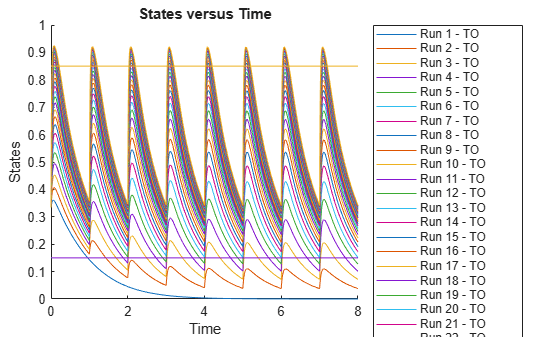Add observable object to SimBiology model

## Syntax

``obsObj = addobservable(modelObj,obsName,obsExpression)``
``obsObj = addobservable(modelObj,obsName,obsExpression,Name,Value)``

## Description

example

````obsObj = addobservable(modelObj,obsName,obsExpression)` adds an `observable` object to a SimBiology® model `modelObj`. The inputs `obsName` and `obsExpression` are the observable object name and its expression, respectively.```

example

````obsObj = addobservable(modelObj,obsName,obsExpression,Name,Value)` sets the property values of `obsObj` using one or more name-value pair arguments. `Name` is the property name and `Value` is the corresponding value `Name` must appear inside quotes. You can specify several name and value pair arguments in any order as `Name1,Value1,...,NameN,ValueN`. For a list of properties, see observable object properties.```

## Examples

collapse all

`sbioloadproject tmdd_with_TO.sbproj`

Set the target occupancy (`TO`) as a response.

```cs = getconfigset(m1); cs.RuntimeOptions.StatesToLog = 'TO';```

Get the dosing information.

`d = getdose(m1,'Daily Dose');`

Add two scalar observables that represent the safety and efficacy thresholds for `TO`. In this example, suppose that any `TO` value above 0.85 is unsafe, and any `TO` value below 0.15 has no efficacy.

```safetyTO = addobservable(m1,'SafetyThreshold','0.85','Unit','dimensionless'); efficacyTO = addobservable(m1,'EfficacyThreshold','0.15','Unit','dimensionless');```

Scan over different dose amounts using a `SimBiology.Scenarios` object. To do so, first parameterize the `Amount` property of the dose. Then vary the corresponding parameter value using the `Scenarios` object.

```amountParam = addparameter(m1,'AmountParam','Units',d.AmountUnits); d.Amount = 'AmountParam'; d.Active = 1; doseSamples = SimBiology.Scenarios('AmountParam',linspace(0,300,31));```

Create a `SimFunction` to simulate the model. Set `TO` and two thresholds (observables) as the simulation outputs.

```% Suppress informational warnings that are issued during simulation. warning('off','SimBiology:SimFunction:DOSES_NOT_EMPTY'); f = createSimFunction(m1,doseSamples,{'TO','SafetyThreshold','EfficacyThreshold'},d)```
```f = SimFunction Parameters: Name Value Type Units _______________ _____ _____________ ____________ {'AmountParam'} 1 {'parameter'} {'nanomole'} Observables: Name Type Units _____________________ ______________ _________________ {'TO' } {'parameter' } {'dimensionless'} {'SafetyThreshold' } {'observable'} {'dimensionless'} {'EfficacyThreshold'} {'observable'} {'dimensionless'} Dosed: TargetName TargetDimension Amount AmountValue AmountUnits _______________ ___________________________________ _______________ ___________ ____________ {'Plasma.Drug'} {'Amount (e.g., mole or molecule)'} {'AmountParam'} 1 {'nanomole'} ```
`warning('on','SimBiology:SimFunction:DOSES_NOT_EMPTY');`

Simulate the model using the dose amounts generated by the `Scenarios` object. In this case, the object generates 31 different doses; hence the model is simulated 31 times and generates a `SimData` array.

```doseTable = getTable(d); sd = f(doseSamples,cs.StopTime,doseTable)```
``` SimBiology Simulation Data Array: 31-by-1 ModelName: TMDD Logged Data: Species: 0 Compartment: 0 Parameter: 1 Sensitivity: 0 Observable: 2 ```

Plot the simulation results. The two horizontal lines represent the safety and efficacy thresholds. Note that certain `TO` responses either exceed the safety threshold or dip below the efficacy threshold.

`sbioplot(sd);`Postprocess the simulation results. Find out which dose amounts are effective, corresponding to the `TO` responses within the safety and efficacy thresholds. To do so, add an observable expression to the simulaton data.

```% Suppress informational warnings that are issued during simulation. warning('off','SimBiology:sbservices:SB_DIMANALYSISNOTDONE_MATLABFCN_UCON'); newSD = addobservable(sd,'stat1','max(TO) < 0.85 & min(TO) > 0.15','Units','dimensionless')```
``` SimBiology Simulation Data Array: 31-by-1 ModelName: TMDD Logged Data: Species: 0 Compartment: 0 Parameter: 1 Sensitivity: 0 Observable: 3 ```

The addobservable function evaluates the new observable expression for each `SimData` in `sd` and returns the evaluated results as a new `SimData` array. `newSD` has three observables. The first two correspond to the safety and efficacy thresholds. The third is the added observable (`stat1`).

SimBiology stores the observable results in two different properties of a `SimData` object. If the results are scalar-valued, they are stored in `SimData.ScalarObservables`. Otherwise, they are stored in `SimData.VectorObservables`. In this example, the `stat1` observable expression is scalar-valued.

Extract the scalar observable values and plot them against the dose amounts.

```scalarObs = vertcat(newSD.ScalarObservables); doseAmounts = generate(doseSamples); plot(doseAmounts.AmountParam,scalarObs.stat1,'o','MarkerFaceColor','b')```The plot shows that dose amounts ranging from 50 to 180 nanomoles provide `TO` responses that lie within the target efficacy and safety thresholds.

You can update the observable expression with different threshold amounts. The function recalculates the expression and returns the results in a new `SimData` object array.

`newSD2 = updateobservable(newSD,'stat1','max(TO) < 0.75 & min(TO) > 0.30');`

Rename the observable expression. The function renames the observable, updates any expressions that reference the renamed observable (if applicable), and returns the results in a new `SimData` object array.

`newSD3 = renameobservable(newSD2,'stat1','EffectiveDose');`

Restore the warning settings.

`warning('on','SimBiology:sbservices:SB_DIMANALYSISNOTDONE_MATLABFCN_UCON');`

## Input Arguments

collapse all

SimBiology model, specified as a SimBiology `model object`.

Name of the observable object, specified as a character vector or string.

The name

• Cannot contain the characters `[ ]`, `->`, or `<->`.

• Cannot be empty, the word time, the word null, or all whitespace.

• Must be unique in a model, meaning no observable object can have the same name as another observable, species, compartment, parameter, reaction, variant, or dose in the model.

For details, see Guidelines for Naming Model Components.

Example: `'AUC_obs'`

Data Types: `char` | `string`

Expression of the observable object, specified as a character vector or string.

Example: `'trapz(time,drug)'`

Data Types: `char` | `string`

## Output Arguments

collapse all

Observable object, returned as an `observable` object.